Welcome to my annual Pi Day post. Every year on March 14th (written 3/14 in the US), geeky mathematicians and their friends celebrate "all things pi-related" because 3.14 is the three-decimal approximation to pi.

Pi is a mathematical constant that never changes. Pi is the same value today as it was in ancient Babylon and Greece. The timeless constancy of pi is a comforting presence in a world of rapid change.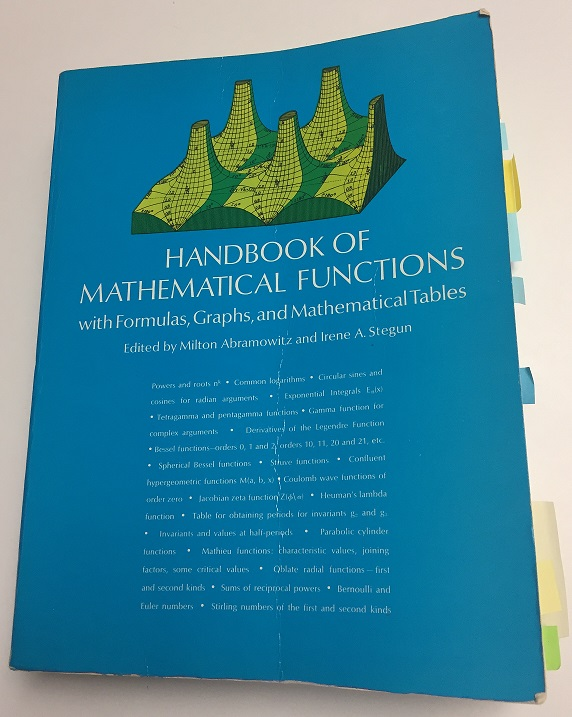But even though the value of pi does not change, our knowledge about pi does change and grow. I was reminded of this recently when I opened my worn copy of the Handbook of Mathematical Functions (more commonly known as "Abramowitz and Stegun," the names of its editors). When the 1,046-page Handbook was published in 1964, it was the premier reference volume for applied mathematicians and mathematical scientists. Interestingly, pi is not even listed in the index! It does appear on p. 3 under "Mathematical Constants," which gives a 25-digit approximation of many mathematical constants such as pi, e, and sqrt(2).

### How to define pi?

Fast forward to the age of the internet. In 2010, the Handbook was transformed into an expanded online, searchable, interactive web site. The new Handbook is called The NIST Digital Library of Mathematical Functions. This is very exciting because the Handbook is now available (for free!) to everyone!

If you search for pi in the online Digital Library, you find that the editors chose to define pi as the value of the integral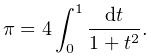This seems to be a strange way to define pi. Pi is the ratio of the circumference and diameter of a circle, and upon first glance that formula doesn't seem related to a circle. A more geometric choice would be an integrand such as sqrt(1 + t2), which connects pi to the area under the unit circle.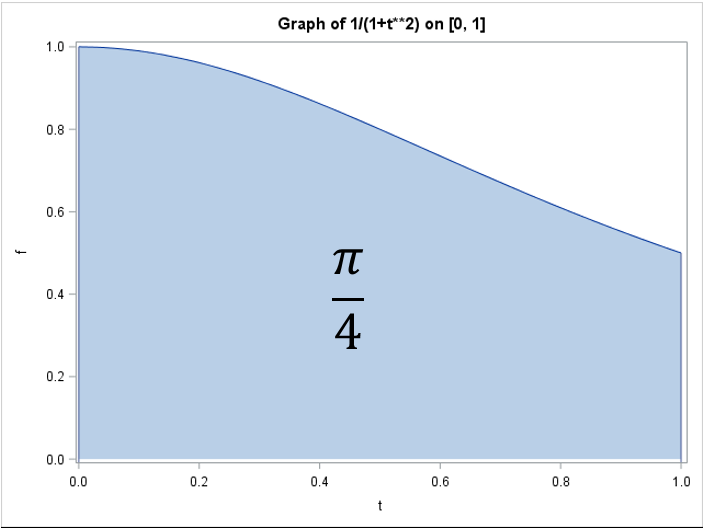Of course, the integral in the Digital Library is equal to pi, but it is not obvious. You might recall from calculus that the antiderivative of 1/(1+t2) is arctan(t). Therefore the expression is just a complicated way to write 4 arctan(1). Ah! This makes more sense because arctan(1) is equal to π/4. In fact, before SAS introduced the CONSTANT function, SAS programmers used to define pi by using the computation pi = 4*ATAN(1). Nevertheless, I think expressing arctan(1) as an integral is unnecessarily obtuse.

### Using the Cauchy distribution to define pi

I am not enamored with the editors' choice of an integral to define pi, but if I were to use that integrand to define pi, I would use a variation that has applications in probability and statistics. Statisticians sometimes use the Cauchy distribution, which is a fat-tailed distribution that has the interesting mathematical property that the distribution has no mean (expected) value! (Mathematicians say that "the first moment does not exist.") Researchers in robust statistical methods sometimes use Cauchy-distributed errors to generate extreme outliers in simulated data.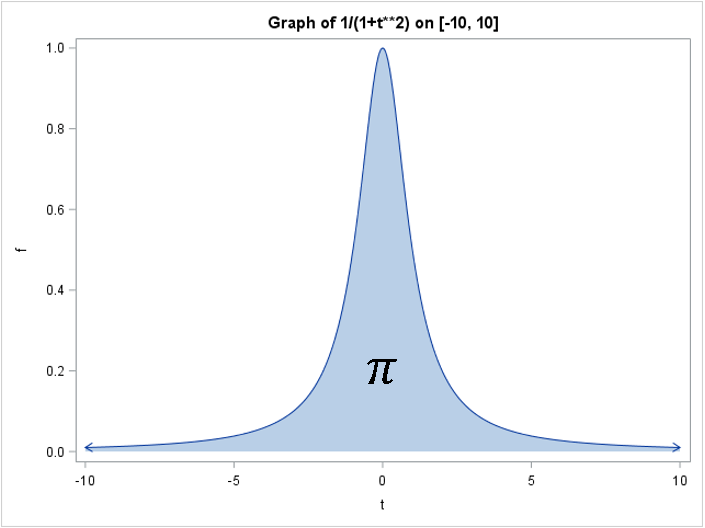The Cauchy probability density function (PDF) is 1/π 1/(1+t2), which means that the integral of the PDF on the interval [-∞, ∞] is 1. Equivalently, the integral of 1/(1+t2) on the interval [-∞, ∞] is π: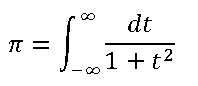This definition of pi seems more natural than the integral on [0, 1]. I could make other suggestions (such as the integral of arccos on [-1, 1]), but I think I'll stop here.

The purpose of this post is to celebrate pi, which is so ubiquitous and important that it can be defined in numerous ways. A secondary purpose is to highlight the availability of the NIST Digital Library of Mathematical Functions, which is an online successor of the venerable Handbook of Mathematical Functions. I am thrilled with the availability of this amazing resource, regardless of how they define pi!

To complete this Pi Day post, I leave you with a pi-ku. A pi-ku is like a haiku, but each line contains syllables the number of syllables in the decimal expansion of pi. A common structure for a pi-ku is 3-1-4. The following pi-ku celebrates the new Digital Library:

Handbook of
Math
Functions? Online!
Tags pi day
Share1.